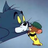V2EX = way to explore
V2EX 是一个关于分享和探索的地方

V2EX  ›  数学

# 如何证明证明鲍勃抛出的正面数比爱丽丝抛出的正面数多的概率为 1/2 ？

huzhikuizainali · 216 天前 · 544 次点击

An alternative solution is to argue that if Alice and Bob are tied after 2n tosses, they are equally likely to win. If they are not tied, then their scores differ by at least 2, and toss 2n+1 will not change the final outcome. This argument may also be expressed algebraically by using the total probability theorem. Let B . Let X be the event that after each has tossed n of their coins, Bob has more heads than Alice, let Y be the event that under the same conditions, Alice has more heads than Bob, and let Z be the event that they have the same number of heads. Since the coins are fair, we have P(X) = P(Y ), and also P(Z) = 1 − P(X) − P(Y ). Furthermore, we see that P(B | X) = 1, P(B | Y ) = 0, P(B|Z)=0.5

Now we have, using the total probability theorem,

P(B) = P(X) · P(B | X) + P(Y ) · P(B | Y ) + P(Z) · P(B | Z)

    = P(X) + 0.5· P(Z)
= ( P(X) + P(Y ) + P(Z) ) · 0.5
=0.5


as required.

7 条回复1 cosette      216 天前 P(B|Z)表示“在双方各自抛完 n 次硬币后，双方正面次数相等的情况下，Bob 抛完最后一次之后，总正面数量多于 Alice 的概率”，就是“前 n 次正面次数相等的情况下，Bob 最后一次得到正面的概率”，因为每一次抛硬币都是独立事件，所以 P(B|Z)就是单独抛一枚硬币得到正面的概率，是 0.5 。这个 0.5 的概率是在事件 Z“前 n 次得到相同正面数”的前提下的条件概率，并不是在全部样本空间中的概率（全概率）。2 huzhikuizainali   OP   216 天前 @cosette 谢谢回复。首先我发现英文答案 Let B 后面少了一些内容，补充如下：Let B "be the event that Bob tosses more heads" 其次，“If they are not tied, then their scores differ by at least 2”--------如果我没理解错，这个结论依赖于如下积分规则，“head 得 1 分，tail 得-1 分”。如果是 head 得 1 分，tail 得 0 分，则这个结论就不成立了。对吧。而且 P(B | X) = 1 也依赖于此，既前 n 次如果 Bob 得分领先，那么 Bob 即便第 n+1 次投出 tail ，最终 Bob 还是赢过 Alice ，所以概率是 1 。对吧？再次，如果这个问题稍稍改动，两人各投 n 次，Bob 正面赢过 Alice 的概率是多少？这怎么计算？是要先分类后计算概率？例如：计算 n-1 次两人得分一致，只有一次 Bob 是头，Alice 是尾。 再计算 n-2 次两人得分一致，有两次 Bob 是头，Alice 是尾。穷举计算？3 cosette      215 天前 如果投掷相同数量的硬币，那么可以对样本空间进行一个划分：Bob 的正面更多，Bob 的正面更少和 Bob 的正面数与 Alice 一样，考虑到“Bob 的正面多”和“Alice 的正面多”两组事件是对称的，概率应该相等，所以可以记为 P(B)=(1-P(S))/2 ，其中，P(S)表示在全空间中两者 Heads 数相等的概率。当然也可以在全样本空间中，利用古典概型做排列组合计算，Alice 和 Bob 各自的样本空间是 n 次抛硬币的结果构成的全排列空间，全样本空间可以用两个样本空间的笛卡尔积表示。分步计算使用的是排列组合的乘法原理，针对古典概型问题来说。4 cosette      215 天前 @cosette 更正一点，不是全排列，就是排列，n 个 Heads 和 n 个 Tail 中选 n 个出来做一个排列5 huzhikuizainali   OP   215 天前 @cosette 谢谢你再次详细的解答。此回复针对 3 楼。所以可以记为 P(B)=(1-P(S))/2 ，其中，P(S)表示在全空间中两者 Heads 数相等的概率。--------其实 P(S)对应的就是题目答案中所说的 P(X)=P(Y)的情况。但是即便如此似乎还是无法求得 P(S)的具体数值吧？因此 P(B)还是无法根据这个公式代入 P(S)求出来吧？6 cosette      214 天前1 @huzhikuizainali P(S) 概率直接求就行了。设$P_i$表示 Alice 或者 Bob 抛完 n 枚硬币得到 i 个 Heads 的概率，则有$P_i=\frac{C_n^i}{2^n}$，那么$P(S)=\sum_{i=0}^{n}{P_i^2}$。7 huzhikuizainali   OP   214 天前 @cosette 谢谢回复。我明白了。